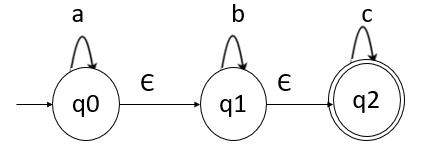# What is an acceptance of language by NFA with Epsilon?

The language L accepted by Non-deterministic finite automata (NFA) with ε, denoted by M= (Q, Σ, 𝛿, q0, F) and can be defined as follows −

Let M= (Q, Σ, 𝛿, q0, F) be a NFA with ε

Where,

• Q is a set of states
• Σ is input set
• δ is a transition function from Q x { Σ U ε } to 2Q
• q0 is start state
• F is a final state

The string w in L accepted by NFA can be represented as follows −

L(M)={w| w ∈ Σ* and 𝛿 transition for w from q0 reaches to F}

## Example

Construct NFA with epsilon which accepts a language consisting the strings of any number of a’s followed by any number of b’s followed by any number of c’s

## Solution

Here, any number of a’s or b’s or c’s means that there can be zero or more a’s followed by zero, more number of b’s followed by zero or more c’s.

Hence NFA with epsilon can be as follows −Normally, epsilon is not shown in the input string.

The NFA with epsilon is as follows −

M = ({q0,q1,q2},{a,b}, 𝛿, q0,q2})

## Explanation

• Step 1 − q0 is the initial state q0 on ‘a’ goes to q0 itself, and on epsilon transition q0 goes to q1.
• Step 2 − q1 on ‘b’ goes to q1 itself and on epsilon transition it goes to q2.
• Step 3 − q2 on ‘c’ goes to q2 itself which is the final state.

The transition table is as follows −

State\ input symbolabCε
q0q0--q1
q1-q1-q2
q2--q2-

Consider the string aabbcc as given below −

δ(q0,aabbcc) |- δ(q0,abbcc)
|- δ(q0,bbcc)
|- δ(q0, εbbcc)
|- δ(q1,bbcc)
|- δ(q1,bcc)
|- δ(q1,cc)
|- δ(q1, εcc)
|- δ(q2,cc)
|- δ(q2,c)
|- δ(q2, ε)

Thus, we reach the accept state, after scanning the compute input string.